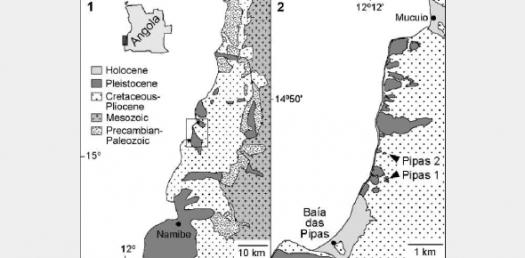# Book M Ch 1 Modified

18 Questions | Total Attempts: 129Settings.

• 1.
Occurs when an object speeds up, slows down, or turns.
• A.

Inertia

• B.

Acceleration

• 2.
Total distance traveled divided by the total time it took to travel that distance.
• A.

Average speed

• B.

Velocity

• 3.
The speed of an object at one instant of time.
• A.

Average speed

• B.

Momentum

• 4.
The measure of how difficult it is to stop a moving object.
• A.

Average speed

• B.

Momentum

• 5.
The speed and direction of a moving object
• A.

Velocity

• B.

Inertia

• 6.
The distance and direction from a starting point to a stopping point
• A.

Acceleration

• B.

Displacement

• 7.
Calculate the speed of a swimmer who swam 150m in 88s. Round to the nearest 10th.  ________ m/s. (Put the numeric value only, not the m/s).  You may use your calculator.
• 8.
A girl walked 200 meters north, 100 meters east,and 200 meters south. What is the total distance she traveled in meters? Put the numeric value only.
• 9.
A girl walked 200 meters north, 100 meters east,and 200 meters south. What was her displacement?  _____m East.
• 10.
An object has a speed of 2.00 m/s. Three seconds later it is going 8.00 m/s. What average acceleration did it experience?   ______ m/s 2. You may use your calculator.
• 11.
What is the SI unit used for distance?
• A.

Kg

• B.

M

• 12.
What is the SI unit for mass?
• A.

Kg

• B.

M

• 13.
How is acceleration expressed?
• A.

M/s

• B.

M/s2

• 14.
What does a horizontal line on a distance/time graph represent?
• A.

0 speed (stopped)

• B.

No change in accleration

• 15.
What does a line sloping upward mean in a Velocity/time graph mean?
• A.

Positive acceleration

• B.

Negative acceleration

• 16.
The momentum of a falling leaf is ___ than the momentum of a falling pinecone.
• A.

Less than

• B.

Greater than

• 17.
A 50 kg object moves with a velocity of 10 m/s. What is its momentum?  _______ kg x m/s.  (Write the numeric value only). You may use your calculator.
• 18.
Choose one of the following to write an essay on. -Why do we say that a merry-go-round is accelerating if it is going a constant speed? -If you hear that a tornado in a town near you is traveling 25 km/h, can you determine if you should seek shelter? -Why would an airplane's displacement be nearly the same as the distance it traveled?
Related Topics